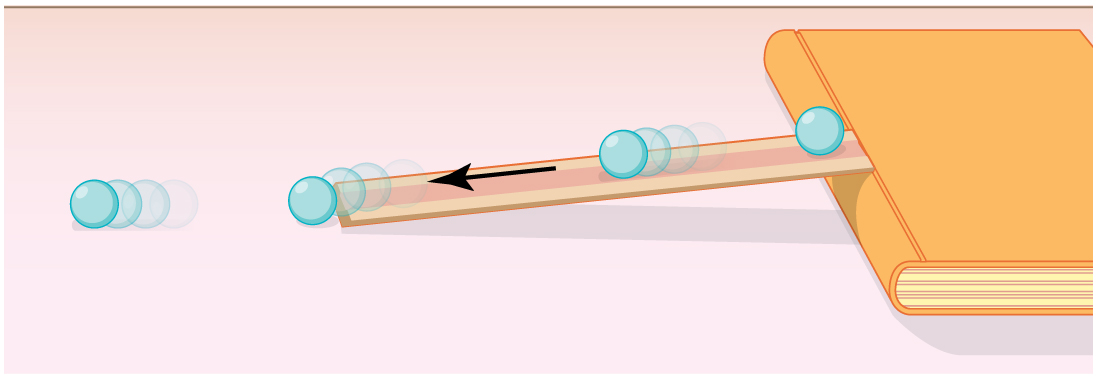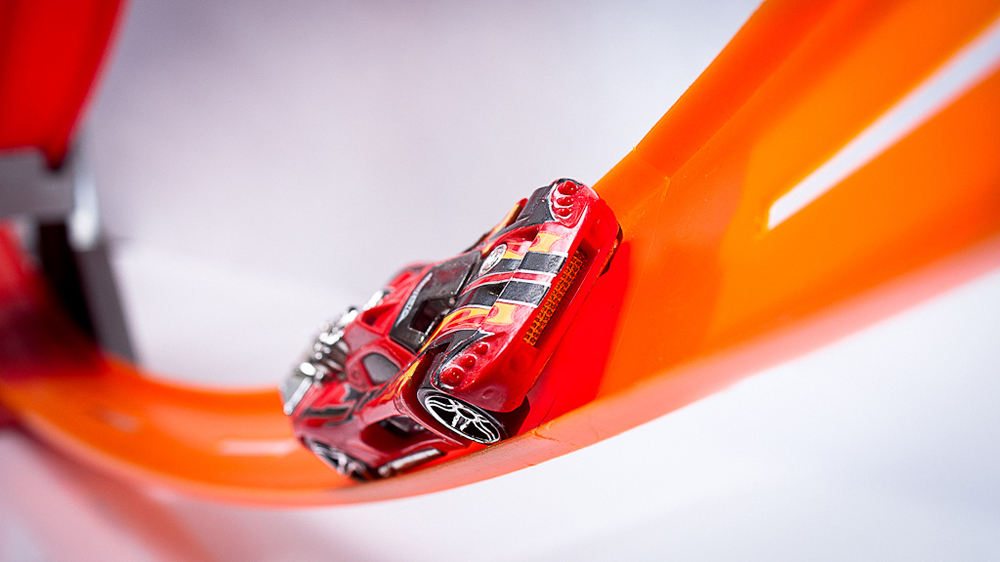# 7.3 Gravitational potential energy  (Page 4/5)

 Page 4 / 5

We have seen that work done by or against the gravitational force depends only on the starting and ending points, and not on the path between, allowing us to define the simplifying concept of gravitational potential energy. We can do the same thing for a few other forces, and we will see that this leads to a formal definition of the law of conservation of energy.

## Making connections: take-home investigation—converting potential to kinetic energy

One can study the conversion of gravitational potential energy into kinetic energy in this experiment. On a smooth, level surface, use a ruler of the kind that has a groove running along its length and a book to make an incline (see [link] ). Place a marble at the 10-cm position on the ruler and let it roll down the ruler. When it hits the level surface, measure the time it takes to roll one meter. Now place the marble at the 20-cm and the 30-cm positions and again measure the times it takes to roll 1 m on the level surface. Find the velocity of the marble on the level surface for all three positions. Plot velocity squared versus the distance traveled by the marble. What is the shape of each plot? If the shape is a straight line, the plot shows that the marble’s kinetic energy at the bottom is proportional to its potential energy at the release point.A marble rolls down a ruler, and its speed on the level surface is measured.

## Section summary

• Work done against gravity in lifting an object becomes potential energy of the object-Earth system.
• The change in gravitational potential energy, $\Delta {\text{PE}}_{\text{g}}$ , is ${\text{ΔPE}}_{g}=\text{mgh}$ , with $h$ being the increase in height and $g$ the acceleration due to gravity.
• The gravitational potential energy of an object near Earth’s surface is due to its position in the mass-Earth system. Only differences in gravitational potential energy, ${\text{ΔPE}}_{g}$ , have physical significance.
• As an object descends without friction, its gravitational potential energy changes into kinetic energy corresponding to increasing speed, so that $\text{ΔKE}\text{= −}{\text{ΔPE}}_{\text{g}}$ .

## Conceptual questions

In [link] , we calculated the final speed of a roller coaster that descended 20 m in height and had an initial speed of 5 m/s downhill. Suppose the roller coaster had had an initial speed of 5 m/s uphill instead, and it coasted uphill, stopped, and then rolled back down to a final point 20 m below the start. We would find in that case that it had the same final speed. Explain in terms of conservation of energy.

Does the work you do on a book when you lift it onto a shelf depend on the path taken? On the time taken? On the height of the shelf? On the mass of the book?

## Problems&Exercises

A hydroelectric power facility (see [link] ) converts the gravitational potential energy of water behind a dam to electric energy. (a) What is the gravitational potential energy relative to the generators of a lake of volume $\text{50}\text{.}0 k{\text{m}}^{3}$ ( $\text{mass}=5\text{.}\text{00}×{\text{10}}^{\text{13}}\phantom{\rule{0.25em}{0ex}}\text{kg}\right)$ , given that the lake has an average height of 40.0 m above the generators? (b) Compare this with the energy stored in a 9-megaton fusion bomb.

(a) $1\text{.}\text{96}×{\text{10}}^{\text{16}}\phantom{\rule{0.25em}{0ex}}\text{J}$

(b) The ratio of gravitational potential energy in the lake to the energy stored in the bomb is 0.52. That is, the energy stored in the lake is approximately half that in a 9-megaton fusion bomb.

(a) How much gravitational potential energy (relative to the ground on which it is built) is stored in the Great Pyramid of Cheops, given that its mass is about and its center of mass is 36.5 m above the surrounding ground? (b) How does this energy compare with the daily food intake of a person?

Suppose a 350-g kookaburra (a large kingfisher bird) picks up a 75-g snake and raises it 2.5 m from the ground to a branch. (a) How much work did the bird do on the snake? (b) How much work did it do to raise its own center of mass to the branch?

(a) 1.8 J

(b) 8.6 J

In [link] , we found that the speed of a roller coaster that had descended 20.0 m was only slightly greater when it had an initial speed of 5.00 m/s than when it started from rest. This implies that . Confirm this statement by taking the ratio of $\text{Δ}\text{PE}$ to ${\text{KE}}_{\text{i}}$ . (Note that mass cancels.)

A 100-g toy car is propelled by a compressed spring that starts it moving. The car follows the curved track in [link] . Show that the final speed of the toy car is 0.687 m/s if its initial speed is 2.00 m/s and it coasts up the frictionless slope, gaining 0.180 m in altitude.A toy car moves up a sloped track. (credit: Leszek Leszczynski, Flickr)
${v}_{f}=\sqrt{2\text{gh}+{{v}_{0}}^{2}}=\sqrt{2\left(\text{9.80 m}{\text{/s}}^{2}\right)\left(-0\text{.180 m}\right)+\left(2\text{.00 m/s}{\right)}^{2}}=0\text{.687 m/s}$

In a downhill ski race, surprisingly, little advantage is gained by getting a running start. (This is because the initial kinetic energy is small compared with the gain in gravitational potential energy on even small hills.) To demonstrate this, find the final speed and the time taken for a skier who skies 70.0 m along a $\text{30º}$ slope neglecting friction: (a) Starting from rest. (b) Starting with an initial speed of 2.50 m/s. (c) Does the answer surprise you? Discuss why it is still advantageous to get a running start in very competitive events.

what is physics
what are the basic of physics
faith
tree physical properties of heat
tree is a type of organism that grows very tall and have a wood trunk and branches with leaves... how is that related to heat? what did you smoke man?
what are the uses of dimensional analysis
Dimensional Analysis. The study of relationships between physical quantities with the help of their dimensions and units of measurements is called dimensional analysis. We use dimensional analysis in order to convert a unit from one form to another.
Emmanuel
meaning of OE and making of the subscript nc
Negash
kinetic functional force
what is a principal wave?
A wave the movement of particles on rest position transferring energy from one place to another
Gabche
not wave. i need to know principal wave or waves.
Haider
principle wave is a superposition of wave when two or more waves meet at a point , whose amplitude is the algebraic sum of the amplitude of the waves
kindly define principal wave not principle wave (principle of super position) if u can understand my question
Haider
what is a model?
hi
Muhanned
why are electros emitted only when the frequency of the incident radiation is greater than a certain value
b/c u have to know that for emission of electron need specific amount of energy which are gain by electron for emission . if incident rays have that amount of energy electron can be emitted, otherwise no way.
Nazir
Nazir
what is ohm's law
states that electric current in a given metallic conductor is directly proportional to the potential difference applied between its end, provided that the temperature of the conductor and other physical factors such as length and cross-sectional area remains constant. mathematically V=IR
ANIEFIOK
hi
Gundala
A body travelling at a velocity of 30ms^-1 in a straight line is brought to rest by application of brakes. if it covers a distance of 100m during this period, find the retardation.
just use v^2-u^2=2as
Gundala
how often does electrolyte emits?
alhassan
just use +€^3.7°√π%-4¢•∆¥%
v^2-u^2=2as v=0,u=30,s=100 -30^2=2a*100 -900=200a a=-900/200 a=-4.5m/s^2
akinyemi
what's acceleration
The change in position of an object with respect to time
Mfizi
Acceleration is velocity all over time
Pamilerin
hi
Stephen
It's not It's the change of velocity relative to time
Laura
Velocity is the change of position relative to time
Laura
acceleration it is the rate of change in velocity with time
Stephen
acceleration is change in velocity per rate of time
Noara
what is ohm's law
Stephen
Ohm's law is related to resistance by which volatge is the multiplication of current and resistance ( U=RI)
Laura
acceleration is the rate of change. of displacement with time.
the rate of change of velocity is called acceleration
Asma
how i don understand
how do I access the Multiple Choice Questions? the button never works and the essay one doesn't either
How do you determine the magnitude of force
mass × acceleration OR Work done ÷ distance
SeemaByByBy OpenStaxBy Ellie BanfieldBy OpenStaxBy OpenStaxBy Madison ChristianBy Nick SwainBy Briana KnowltonBy Janet ForresterBy Jazzycazz JacksonBy OpenStax